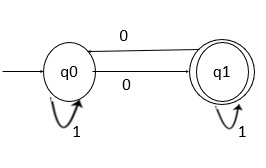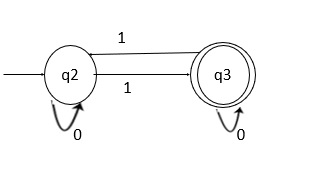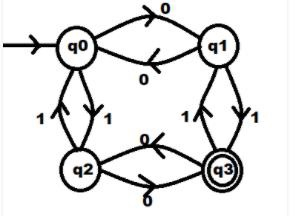# C Program to construct DFA accepting odd numbers of 0s and 1s

Construct deterministic finite automata (DFA) for the language L = { w : w has odd number of 0’s and w has odd number of 1’s},over the alphabet Σ = {0, 1}.

Example

0111,010101,01110011 is an accepted string, because those strings have an odd number of 0’s and an odd number of 1’s.

For the given language we will need four states to draw the main DFA which will read odd no. of 0s and 1s. We can also draw it by merging the two DFAs in which one will accept only an odd number of 0s and one accepts an odd number of 1s.

Follow the steps given below to construct a DFA for the language L = { w : w has odd number of 0’s and w has odd number of 1’s},over the alphabet Σ = {0, 1} −

Step 1 − For an odd number of 0’s.Step 2 − For an odd number of 1’s.Step 3 − Combining step 1 and step 2, we will get the final result.## Explanation

• Let’s take the input 01110011 now first q0 will start and on 0 input, it will go to state q1.

• Now we need to give input 1, then it will go to state q3.

• Now on q3 again, we give input 1. It will go to state q1 then again 1 and it will reach at q3 after reading 0111.

• Now give the input 0, it will go to state q2 then again on input 0 it will come on state q3.

• Now again when we give 1 as an input, it will go to state q2. Then finally, on getting 1 input on state q2, it will reach state q3 which is the final state.

So, the string is accepted.

## Example

Following is the C program to construct DFA for the language L = { w : w has odd number of 0’s and w has odd number of 1’s},over the alphabet Σ = {0, 1} −

Live Demo

#include <stdio.h>
int EE=0, OE=1, OO=2, EO=3; // DFA states
int state = 0; // initial DFA state
char input;
int main(void) {
printf("Enter a string of 0s and 1s: ");
while (1) {
scanf("%c", &input);
if (input == '') // if end-of-line exit loop
break;
if ( (input != '0') && (input != '1') ) { // invalid input printf("Invalid input: program terminating");
break;
}
if (state==0) {
// input is either '0' or '1' state = (input == '0') ? OE : EO;
}
else if(state==1) {
state = (input == '0') ? EE : OO;
}
else if (state==2) {
state = (input == '0') ? EO : OE;
} else {
state = (input == '0') ? OO : EE;
}
};
if (input == '') {
if (state == OO)
printf("Input accepted");
else
printf("Input rejected");
}
return 0;
}

## Output

When we execute the above program, we get the following output −

Run 1:
Enter a string of 0s and 1s: 101010
Input accepted
Run 2:
Enter a string of 0s and 1s: 10011100
Input rejected